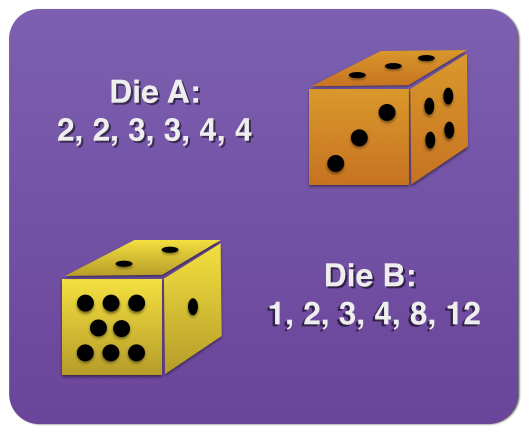# Perfectly Squared Dice

Probability Level 2Two strange six-sided dice are numbered on each side as follows:

• Die A: 2, 2, 3, 3, 4, 4
• Die B: 1, 2, 3, 4, 8, 12

If one were to roll these dice at the same time and multiply the two resulting values, what is the probability that the product would be a perfect square?

×